Analysis WebNotes
arrow_back arrow_forward

# Class Contents

## Riemann-Stieltjes Integration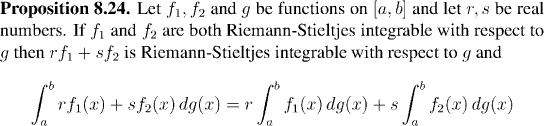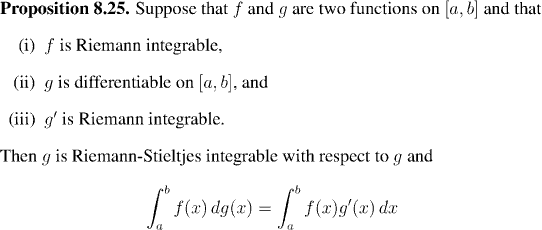Remark:
We can regard the last proposition as an extension of Proposition 8.20. If g(x)=x then this is exactly Proposition 8.25, and, like that result, the present proposition relates Riemann-Stieltjes integrals to plain Riemann integrals.
We can use the last few propositions to calculate more complicated examples of Riemann-Stieltjes integrals, by reducing them to Riemann integrals where possible:
Example: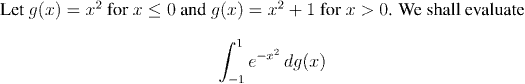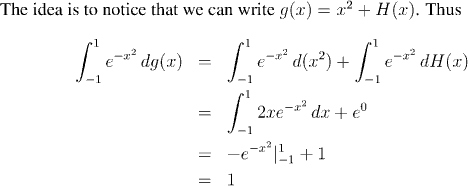Typically weight functions have some behavior which can be described as "smooth" and some behavior which can be described as "discrete", as in the last example. Although it isn't always possible, computations are much easier if you can split the two parts and deal with them separately.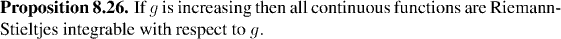Remark:
In view of Proposition 8.22, it follows that if g is any linear combination of two increasing functions, then all continuous functions are Riemann-Stieltjes integrable with respect to g. In particular, if g is the difference of two increasing functions then all continuous functions are Riemann-Stieltjes integrable with respect to g.

Now, it's easy to tell whether or not a function is increasing, but it's not so clear to tell whether or not a function is the difference of two increasing functions, unless you are actually told the increasing functions in question. For example: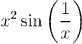can be written as the difference of two increasing functions on [0,1], while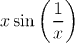cannot be written as the difference of two increasing functions on [0,1].

Fortunately there is a beautiful criterion which allows us to tell whether or not a function can be expressed as the difference of two increasing functions. This is particularly useful for Riemann-Stieltjes integration because other results that hold for Riemann integration (such as the Triangle Inequality) only hold for Riemann-Stieltjes integration when the weight function is of this form.

The condition which tells when a function is the difference of two increasing functions is called bounded variation. Follow the link if you would like to learn more about functions of bounded variation: